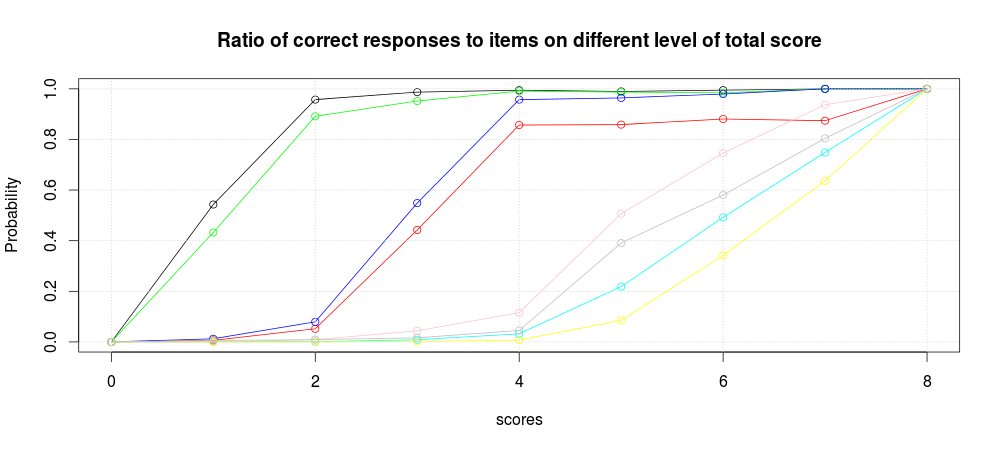# Mobility dataset (8445 samples)```item characteristic curves from all the Mobility dataset (8445 samples)
without fit to a model.
x axis: score (summary)
y axis: probability
``````item characteristic curves from all the dataset (8445 samples) based on ltm model.
```

# Random Sample of Mobility dataset (600 samples)```item characteristic curves from a random sample of Mobility dataset (600 samples)
without fit to a model.
x axis: score (summary)
y axis: probability
``````item characteristic curves from a random sample of Mobility dataset (600 samples) based on ltm model.
```